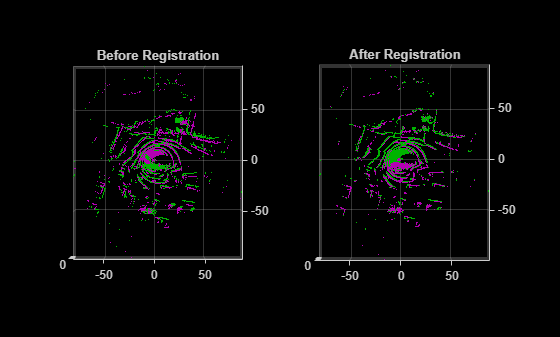# pcregistercorr

Register two point clouds using phase correlation

Since R2020b

## Syntax

``tform = pcregistercorr(moving,fixed,gridSize,gridStep)``
``[tform,rmse] = pcregistercorr(___)``
``[tform,rmse,peak] = pcregistercorr(___)``
``[___] = pcregistercorr(___,Name=Value)``

## Description

example

````tform = pcregistercorr(moving,fixed,gridSize,gridStep)` computes the rigid transformation that registers the moving point cloud `moving`, to the fixed point cloud `fixed`, using an image-based phase correlation algorithm.The function performs registration by first converting both point clouds to a 2-D occupancy grid in the X-Y plane with center at the origin (0,0,0). The occupancy of each grid cell is determined using the Z-coordinate values of points within the grid.```
````[tform,rmse] = pcregistercorr(___)` additionally returns the root mean square error of the Euclidean distance between the aligned point clouds.```
````[tform,rmse,peak] = pcregistercorr(___)` additionally returns the peak correlation value of the phase difference between the two occupancy grids.```
````[___] = pcregistercorr(___,Name=Value)` specifies options using one or more name-value arguments in addition to any combination of arguments from previous syntaxes. For example, `Window=false` sets the `Window` name-value argument to `false` to suppress using windowing .```

## Examples

collapse all

Read data from a Velodyne packet capture (PCAP) file into the workspace.

`veloReader = velodyneFileReader('lidarData_ConstructionRoad.pcap','HDL32E');`

Read a fixed and a moving point cloud from frames of the lidar data.

```frameNumber = 1; skipFrame = 5; fixed = readFrame(veloReader,frameNumber); moving = readFrame(veloReader,frameNumber + skipFrame);```

Find the ground planes for both the moving and the fixed point clouds. Set the maximum distance in meters.

```maxDistance = 0.4; referenceVector = [0 0 1]; groundMoving = pcfitplane(moving,maxDistance,referenceVector); groundFixed = pcfitplane(fixed,maxDistance,referenceVector);```

Transform the point clouds so that their ground planes are parallel to the X-Y plane.

```tformMoving = normalRotation(groundMoving,referenceVector); tformFixed = normalRotation(groundFixed,referenceVector); movingCorrected = pctransform(moving,tformMoving); fixedCorrected = pctransform(fixed,tformFixed);```

Register the moving point cloud against the fixed point cloud. Set the occupancy grid size to 100-by-100 meters and the size of each grid cell to 0.5-by-0.5 meters.

```gridSize = 100; gridStep = 0.5; tform = pcregistercorr(movingCorrected,fixedCorrected,gridSize,gridStep);```

Transform the moving point cloud using the estimated rigid transformation.

```combinedTform = rigidtform3d(tformFixed.A * tformMoving.A * tform.A); movingReg = pctransform(moving,combinedTform);```

Visualize the registration.

```figure subplot(121) pcshowpair(moving,fixed) title('Before Registration') view(2) subplot(122) pcshowpair(movingReg,fixed) title('After Registration') view(2)```## Input Arguments

collapse all

Moving point cloud, specified as a `pointCloud` object.

Fixed point cloud, specified as a `pointCloud` object.

Size of square occupancy grid, specified as a scalar value in world units. The occupancy grid has both width and height equal to this value. The occupancy grid is centered at the origin (0, 0, 0) and the spatial extent of the occupancy grid is [-`gridSize`/2, `gridSize`/2].

Size of each grid cell, specified as a scalar value in world units.

### Name-Value Arguments

Specify optional pairs of arguments as `Name1=Value1,...,NameN=ValueN`, where `Name` is the argument name and `Value` is the corresponding value. Name-value arguments must appear after other arguments, but the order of the pairs does not matter.

Example: `Zlimit=[0 3]` sets the Z-axis lower limit to `0` and the upper limit to `3`.

Before R2021a, use commas to separate each name and value, and enclose `Name` in quotes.

Z-axis limits to compute the occupancy of a grid cell, specified as a vector of the form [zmin zmax], where zmin and zmax are numeric scalars. The function scales points with a Z-axis value from zmin to zmax to probabilities in the range [0, 1]. Values less than zmin are assigned an occupancy value of `0`. Values greater than zmax are assigned an occupancy value of `1`.

Logical to use windowing to suppress spectral leakage effects in the frequency domain, specified as a numeric or logical `0` (`false`) or `1` (`true`). When you set `Window` to `true`, the function uses a Blackman window to increase the stability of registration results. If the common features that will be aligned in the occupancy grids are oriented along the edges, then setting `Window` to `false` could provide superior registration results.

## Output Arguments

collapse all

Rigid transformation, returned as a `rigidtform3d` object. The `rigidtform3d` object describes the rigid 3-D transformation that registers the moving point cloud to the fixed point cloud.

Root mean square error, returned as the Euclidean distance between the aligned point cloud. A low `rmse` value indicates a more accurate registration.

Peak correlation value of the phase difference between the two occupancy grids, returned as a scalar value. A peak value less than `0.03` indicates a poor registration result.

## Tips

• The phase correlation method is best used to register point clouds when the transformation can be described by a translation in the X-Y plane and a rotation around the Z-axis. For example, a ground vehicle with a horizontally mounted lidar moving on a flat surface.

• The phase correlation algorithm expects motion to be exclusively along the X-Y plane, as with the ground plane. If motion is not exactly in the X-Y plane, you can use the `normalRotation` function to transform the point clouds. For example, in vehicular motion, you can reduce the effects of vehicle suspension or surface features such as potholes and speed bumps by using the `normalRotation` function.

• Increasing the size of the occupancy grid increases the computational demands of this function. You can control the size of the occupancy grid by modifying the `gridSize` and `gridStep` arguments.

• If you obtain poor registration results and the `peak` correlation value is less than `0.03`, try setting the `Window` argument to `false`.

 Dimitrievski, Martin, David Van Hamme, Peter Veelaert, and Wilfried Philips. “Robust Matching of Occupancy Maps for Odometry in Autonomous Vehicles.” In Proceedings of the 11th Joint Conference on Computer Vision, Imaging and Computer Graphics Theory and Applications, 626–633. Rome, Italy: SCITEPRESS - Science and Technology Publications, 2016.

 Reddy, B.S., and B.N. Chatterji. “An FFT-Based Technique for Translation, Rotation, and Scale-Invariant Image Registration.” IEEE Transactions on Image Processing 5, no. 8 (August 1996): 1266–71. https://doi.org/10.1109/83.506761.